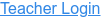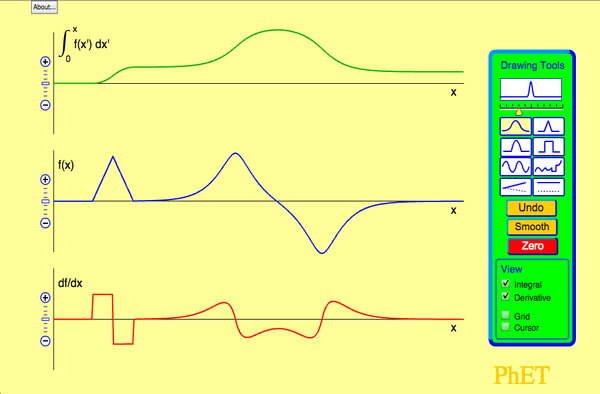• Derivative
• Integral

### Description

Draw a graph of any function and see graphs of its derivative and integral. Don't forget to use the magnify/demagnify controls on the y-axis to adjust the scale.

### Sample Learning Goals

• Given a function sketch, the derivative, or integral curves
• Use the language of calculus to discuss motion
• Explain what the effect of a discontinuity in a function has on the derivative and the integral curves
• Explain the difference between smooth versus piecewise continuous function curve
• Be able to describe in words with illustrations what the derivative and integral functions are.
• Students generalize the idea of a graph [F(x) vs x] or [x(t) vs t] can represent the same thing.
Version 2.08